Graphs in Java: Breadth-First Search (BFS) - Stack Abuse

# Graphs in Java: Breadth-First Search (BFS)### Introduction

Graphs are a convenient way to store certain types of data. The concept was ported from mathematics and appropriated for the needs of computer science.

Due to the fact that many things can be represented as graphs, graph traversal has become a common task, especially used in data science and machine learning.

### Breadth-First Search

Breadth First Search (BFS) visits "layer-by-layer". This means that in a Graph, like shown below, it first visits all the children of the starting node. These children are treated as the "second layer".

Unlike Depth-First Search (DFS), BFS doesn't aggressively go though one branch until it reaches the end, rather when we start the search from a node, it visits all the unvisited neighbors of that node before proceeding to all the unvisited neighbors of another node: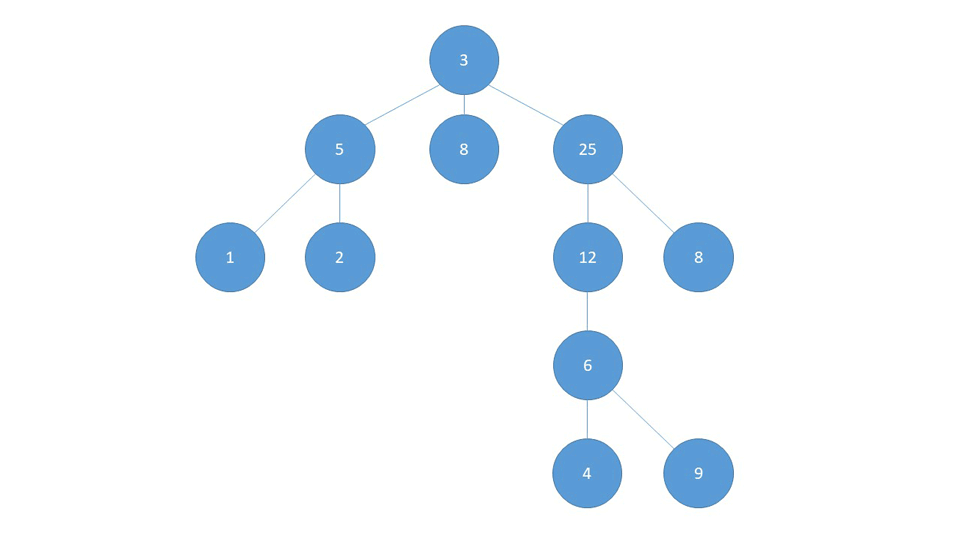### Implementation

We'll use graphs implemented via an adjacency list, like we used for DFS. Also, we need to add the visited attribute alongside the visit() and univisit() methods to our Node class:

public class Node {
int n;
String name;
boolean visited;

Node(int n, String name) {
this.n = n;
this.name = name;
visited = false;
}

void visit() {
visited = true;
}

void unvisit() {
visited = false;
}
}


Now, let's define a Graph:

public class Graph {

// Each node maps to a list of all his neighbors
private HashMap<Node, LinkedList<Node>> adjacencyMap;
private boolean directed;

public Graph(boolean directed) {
this.directed = directed;
adjacencyMap = new HashMap<>();
}

// ...
}


Now, let's add the method addEdge(). We'll use two methods, a helper method and the actual method.

In the helper method, we'll also make a check for possible duplicate edges. Before adding an edge between A and B, we'll first remove it and only then add it. If it existed (we're adding a duplicate edge), it was removed and after adding it again, there's only one.

Though, if it didn't exist, removing a non-existing edge will result in a NullPointerException so we're introducing a temporary copy of the list:

public void addEdgeHelper(Node a, Node b) {
LinkedList<Node> tmp = adjacencyMap.get(a);

if (tmp != null) {
tmp.remove(b);
}
else tmp = new LinkedList<>();
tmp.add(b);
adjacencyMap.put(a,tmp);
}

public void addEdge(Node source, Node destination) {

// We make sure that every used node shows up in our .keySet()
if (!adjacencyMap.keySet().contains(source))
adjacencyMap.put(source, null);

if (!adjacencyMap.keySet().contains(destination))
adjacencyMap.put(destination, null);

addEdgeHelper(source, destination);

// If a graph is undirected, we want to add an edge from destination to source as well
if (!directed) {
addEdgeHelper(destination, source);
}
}


Finally, we'll have the printEdges(), hasEdge() and resetNodesVisited() helper methods, which are pretty straightforward:

public void printEdges() {
for (Node node : adjacencyMap.keySet()) {
System.out.print("The " + node.name + " has an edge towards: ");
for (Node neighbor : adjacencyMap.get(node)) {
System.out.print(neighbor.name + " ");
}
System.out.println();
}
}

public boolean hasEdge(Node source, Node destination) {
return adjacencyMap.containsKey(source) && adjacencyMap.get(source).contains(destination);
}

public void resetNodesVisited(){
for(Node node : adjacencyMap.keySet()){
node.unvisit();
}
}


Let's examine the BFS algorithm on the following undirected graph:

Node 0 has neighbors: 1, 3, 2
Node 1 has neighbors: 0
Node 2 has neighbors: 3, 0
Node 3 has neighbors: 2, 0


We can pick any node to start from, so let's start with 1. We repeat the process of adding and removing nodes from the queue until the queue is empty.

A Queue is a FIFO (first-in-first-out) data structure. It works just like a real-life queue, and so entries are processed (removed from the queue) one by one in the order in which they were added.

This is a very convenient data structure for BFS since we want to process nodes in the order in which we visit them, making sure that we process nodes "closer" to the starting node first.

Since they are added to the queue before any nodes "further" away from the starting node are added to the queue, we know the closer ones will be processed first.

1. We start off by having a queue that contains only node 1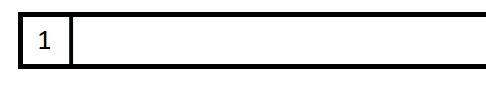1. Remove the first element from the queue, in this case 1, mark it as visited
2. Add all 1's unvisited neighbors to the queue (only 0)

## Git Essentials

Check out this hands-on, practical guide to learning Git, with best-practices and industry-accepted standards. Stop Googling Git commands and actually learn it!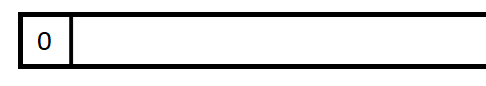1. Remove the first element from the queue, in this case 0, mark it as visited
2. Add all 0's unvisited neighbors to the queue (nodes 3 and 2, 1 has been marked as visited already)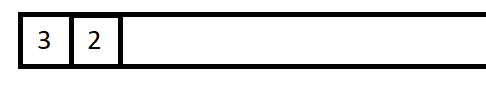1. Remove the first element from the queue, in this case 3, mark it as visited
2. Add all 3's unvisited neighbors to the queue (there are none)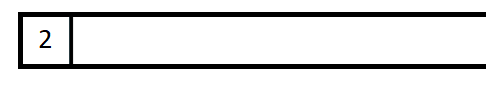1. Remove the first element from the queue, in this case 2, mark it as visited
2. Add all 2's unvisited neighbors to the queue (again, there are none)
3. The queue is now empty, BFS has finished

Our nodes are visited in the 1-0-3-2 order. It should be obvious that set of steps 2-3, 4-5, 6-7, and 8-9 are the same and that step 10 is our loop termination condition. Viewed in this way, it should be easy to write code for our breadthFirstSearch(Node node) method.

There are several types of Queue implementations in Java, but we'll use a LinkedList instead, since it provides all the necessary methods.

We're adding the following method to our Graph class:

void breadthFirstSearch(Node node) {

// Just so we handle receiving an uninitialized Node, otherwise an
// exception will be thrown when we try to add it to queue
if (node == null)
return;

// Creating the queue, and adding the first node (step 1)
LinkedList<Node> queue = new LinkedList<>();
queue.add(node);

while (!queue.isEmpty()) {
Node currentFirst = queue.removeFirst();

// In some cases we might have added a particular node more than once before
// actually visiting that node, so we make sure to check and skip that node if we have
// encountered it before
if (currentFirst.isVisited())
continue;

// Mark the node as visited
currentFirst.visit();
System.out.print(currentFirst.name + " ");

LinkedList<Node> allNeighbors = adjacencyMap.get(currentFirst);

// We have to check whether the list of neighbors is null before proceeding, otherwise
// the for-each loop will throw an exception
if (allNeighbors == null)
continue;

for (Node neighbor : allNeighbors) {
// We only add unvisited neighbors
if (!neighbor.isVisited()) {
queue.add(neighbor);
}
}
}
System.out.println();
}


Now we create our example graph in code and check whether our method works as expected:

public class GraphShow {
public static void main(String[] args) {

Graph graph = new Graph(false);
Node a = new Node(0, "0");
Node b = new Node(1, "1");
Node c = new Node(2, "2");
Node d = new Node(3, "3");
Node e = new Node(4, "4");

graph.addEdge(a,d);
graph.addEdge(a,b);
graph.addEdge(a,c);
graph.addEdge(c,d);

graph.breadthFirstSearch(b);
}
}


Output:

1 0 3 2


If you read the DFS article then you may recall that we encountered a situation where in an unconnected graph, not all of the nodes would be printed out since the algorithm would go through all the nodes it can, and then stop.

The same thing happens with BFS, and this can also happen when graphs are directed, sometimes we can't reach all the nodes. Sometimes this is the behavior we're looking for, but sometimes we want all of the nodes to be visited.

We'll do the same thing as we did in DFS, i.e. we'll keep calling BFS as long as there are any unvisited nodes. We'll make a new breadthFirstSearchModified(Node node) method that does this for us:

void breadthFirstSearchModified(Node node) {
breadthFirstSearch(node);

for (Node n : adjacencyMap.keySet()) {
if (!n.isVisited()) {
breadthFirstSearch(n);
}
}
}

public class GraphShow {
public static void main(String[] args) {

Graph graph = new Graph(false);
Node a = new Node(0, "0");
Node b = new Node(1, "1");
Node c = new Node(2, "2");
Node d = new Node(3, "3");
Node e = new Node(4, "4");

graph.addEdge(a,d);
graph.addEdge(a,b);
graph.addEdge(c,e);

System.out.println("Using the unmodified version of BFS we get:");
graph.breadthFirstSearch(a);

graph.resetNodesVisited();
System.out.println("Using the modified version of BFS we get:");
graph.breadthFirstSearchModified(a);
}
}


Output:

Using the unmodified version of BFS we get:
0 3 1
Using the modified version of BFS we get:
0 3 1
4 2


There is also something called a "bidirectional" BFS search. This is useful when we want to find the shortest path between two vertices (nodes).

This is achieved by simultaneously (in different threads) running a BFS from the starting node and the destination node. This, in theory, finds the shortest path between two nodes twice as fast as running BFS just from the starting node.

Note: Same as with DFS, if we want to go through the neighbors in a particular order (instead of the order in which the edges were added), we can use a PriorityQueue instead of a LinkedList for the list of neighbors.

The code is the same, we just have to implement Comparable and add a compareTo() method to our Node class.

### Conclusion

Graphs are a convenient way to store certain types of data. The concept was ported from mathematics and appropriated for the needs of computer science.

Due to the fact that many things can be represented as graphs, graph traversal has become a common task, especially used in data science and machine learning.

Breadth-First Search is one of the few graph traversal algorithms and visits nodes "layer-by-layer". Unlike Depth-First Search, BFS doesn't aggressively go through one branch until it reaches the end, rather when we start the search from a node, it visits all the unvisited neighbors of that node before proceeding to all the unvisited neighbors of another node.

Last Updated: September 1st, 2020

## Improve your dev skills!

Get tutorials, guides, and dev jobs in your inbox.

No spam ever. Unsubscribe at any time. Read our Privacy Policy.

Olivera PopovićAuthor

LinkedIn: https://rs.linkedin.com/in/227503161
If you need any help - post it in the comments :) That way someone else can reply if I'm busy.

# Prepping for an interview?

• Improve your skills by solving one coding problem every day
• Get the solutions the next morning via email
• Practice on actual problems asked by top companies, like:

# Git Essentials

Check out this hands-on, practical guide to learning Git, with best-practices and industry-accepted standards. Stop Googling Git commands and actually learn it!

© 2013-2021 Stack Abuse. All rights reserved.